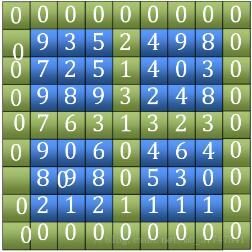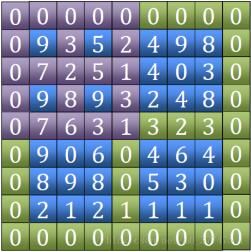# python如何取得二维数组局部峰值25193

0

2020年8月29日00:31:49 评论 71 views

1、找“田”字。包括外围的四条边和中间横竖两条边（图中绿色部分），比较其大小，找到最大值的位置。（图中的7）2、找到田字中最大值后，判断它是不是局部峰值，如果是返回该坐标，如果不是，记录找到相邻四个点中最大值坐标。通过该坐标所在的象限缩小范围，继续比较下一个田字3、当范围缩小到3*3时必定会找到局部峰值（也可能之前就找到了）

```import numpy as np
def max_sit(*n):     #返回最大元素的位置
temp = 0
sit = 0
for i in range(len(n)):
if(n[i]>temp):
temp = n[i]
sit = i
return sit
def dp(s1,s2,e1,e2):
m1 = int((e1-s1)/2)+s1   #row
m2 = int((e2-s1)/2)+s2   #col
nub = e1-s1
temp = 0
sit_row = 0
sit_col = 0
for i in range(nub):
t = max_sit(list[s1][s2+i],     #第一排
list[m1][s2+i],     #中间排
list[e1][s2+i],     #最后排
list[s1+i][s2],     #第一列
list[s1+i][m2],     #中间列
list[s1+i][e2],     #最后列
temp)
if(t==6):
pass
elif(t==0):
temp = list[s1][s2+i]
sit_row = s1
sit_col = s2+i
elif(t==1):
temp = list[m1][s2+i]
sit_row = m1
sit_col = s2+i
elif(t==2):
temp = list[e1][s2+i]
sit_row = e1
sit_col = s2+i
elif(t==3):
temp = list[s1+i][s2]
sit_row = s1+i
sit_row = s2
elif(t==4):
temp = list[s1+i][m2]
sit_row = s1+i
sit_col = m2
elif(t==5):
temp = list[s1+i][e2]
sit_row = s1+i
sit_col = m2
t = max_sit(list[sit_row][sit_col],   #中
list[sit_row-1][sit_col],  #上
list[sit_row+1][sit_col],  #下
list[sit_row][sit_col-1],  #左
list[sit_row][sit_col+1])  #右
if(t==0):
return [sit_row-1,sit_col-1]
elif(t==1):
sit_row-=1
elif(t==2):
sit_row+=1
elif(t==3):
sit_col-=1
elif(t==4):
sit_col+=1
if(sit_row<m1):
e1 = m1
else:
s1 = m1
if(sit_col<m2):
e2 = m2
else:
s2 = m2
return dp(s1,s2,e1,e2)
f = open("demo.txt","r")
list = f.read()
list = list.split("\n")       #对行进行切片
list = ["0 "*len(list)]+list+["0 "*len(list)] #加上下的围墙
for i in range(len(list)):      #对列进行切片
list[i] = list[i].split()
list[i] = ["0"]+list[i]+["0"]    #加左右的围墙
list = np.array(list).astype(np.int32)
row_n = len(list)
col_n = len(list)
ans_sit = dp(0,0,row_n-1,col_n-1)
print("找到峰值点位于：",ans_sit)
print("该峰值点大小为：",list[ans_sit+1,ans_sit+1])
f.close()```

max_sit(*n)函数用于找到多个值中最大值的位置，返回其位置，python的内构的max函数只能返回最大值，所以还是需要自己写，*n表示不定长参数，因为我需要在比较田和十（判断峰值）都用到这个函数

```def max_sit(*n):     #返回最大元素的位置
temp = 0
sit = 0
for i in range(len(n)):
if(n[i]>temp):
temp = n[i]
sit = i
return sit```

dp(s1,s2,e1,e2)函数中四个参数的分别可看为startx，starty，endx，endy。即我们查找范围左上角和右下角的坐标值。

m1,m2分别是row 和col的中间值，也就是田字的中间。

```def dp(s1,s2,e1,e2):
m1 = int((e1-s1)/2)+s1   #row
m2 = int((e2-s1)/2)+s2   #col```

``` for i in range(nub):
t = max_sit(list[s1][s2+i],     #第一排
list[m1][s2+i],     #中间排
list[e1][s2+i],     #最后排
list[s1+i][s2],     #第一列
list[s1+i][m2],     #中间列
list[s1+i][e2],     #最后列
temp)
if(t==6):
pass
elif(t==0):
temp = list[s1][s2+i]
sit_row = s1
sit_col = s2+i
elif(t==1):
temp = list[m1][s2+i]
sit_row = m1
sit_col = s2+i
elif(t==2):
temp = list[e1][s2+i]
sit_row = e1
sit_col = s2+i
elif(t==3):
temp = list[s1+i][s2]
sit_row = s1+i
sit_row = s2
elif(t==4):
temp = list[s1+i][m2]
sit_row = s1+i
sit_row = m2
elif(t==5):
temp = list[s1+i][e2]
sit_row = s1+i
sit_row = m2```

```t = max_sit(list[sit_row][sit_col],   #中
list[sit_row-1][sit_col],  #上
list[sit_row+1][sit_col],  #下
list[sit_row][sit_col-1],  #左
list[sit_row][sit_col+1])  #右
if(t==0):
return [sit_row-1,sit_col-1]
elif(t==1):
sit_row-=1
elif(t==2):
sit_row+=1
elif(t==3):
sit_col-=1
elif(t==4):
sit_col+=1```

``` if(sit_row<m1):
e1 = m1
else:
s1 = m1
if(sit_col<m2):
e2 = m2
else:
s2 = m2

return dp(s1,s2,e1,e2)```

```#!/usr/bin/python3
def dp(n):
temp = (str[n],str[n-9],str[n-1],str[n+1],str[n+9])  #中 上 左 右 下
sit = temp.index(max(temp))
if(sit==0):
return str[n]
elif(sit==1):
return dp(n-9)
elif(sit==2):
return dp(n-1)
elif(sit==3):
return dp(n+1)
else:
return dp(n+9)
f = open("/home/nancy/桌面/demo.txt","r")
list = f.read()
list = list.replace(" ","").split()  #转换为列表
row = len(list)
col = len(list)
str="0"*(col+3)
for x in list:      #加围墙 二维变一维
str+=x+"00"
str+="0"*(col+1)
mid = int(len(str)/2)
print(str,mid)
p = dp(mid)
print (p)
f.close()```

Python接口使用OpenCV的方法

Python变量赋值的步奏详解• 本文由 发表于 2020年8月29日00:31:49
• 转载请务必保留本文链接：https://www.qieseo.com/344324.html

## 初步认识Python中的列表与位运算符

Python列表 List（列表） 是 Python 中使用最频繁的数据类型。 列表可以完成大多数集合类的数据结构实现。它支持字符，数字，字符串甚至可以包含列表（所谓嵌套）。 列表用< >标识。是py...